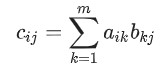12714. 矩阵A×B

• 题目详情
• 详解
• 测评详情1≤ n, m, p ≤100 -10000≤ aij, bij ≤10000

2 3 1 2 3 3 2 1 2 1 1 2 2 3 3

14 14 10 10

14=1×1+2×2+3×3 14=1×1+2×2+3×3 10=3×1+2×2+1×3 10=3×1+2×2+1×3